• 多属性决策模型 通过层次分析模型得到的 权重与属性值相乘 再找最优 层次分析方法 请看上篇博客 例子然后各项指标的重要性创建比较矩阵，通过层次分析法得到各权重。 利用各项指标权值与每个公司对应数据相乘再...
多属性决策模型    通过层次分析模型得到的  权重与属性值相乘     再找最优
层次分析方法    请看上篇博客层次分析法
例子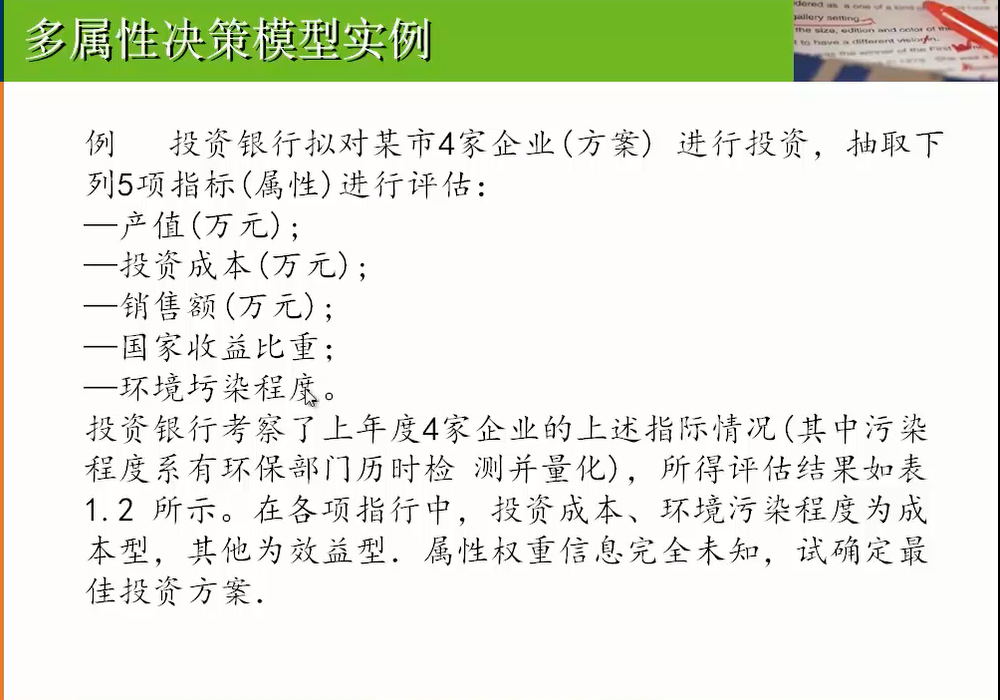然后各项指标的重要性创建比较矩阵，通过层次分析法得到各权重。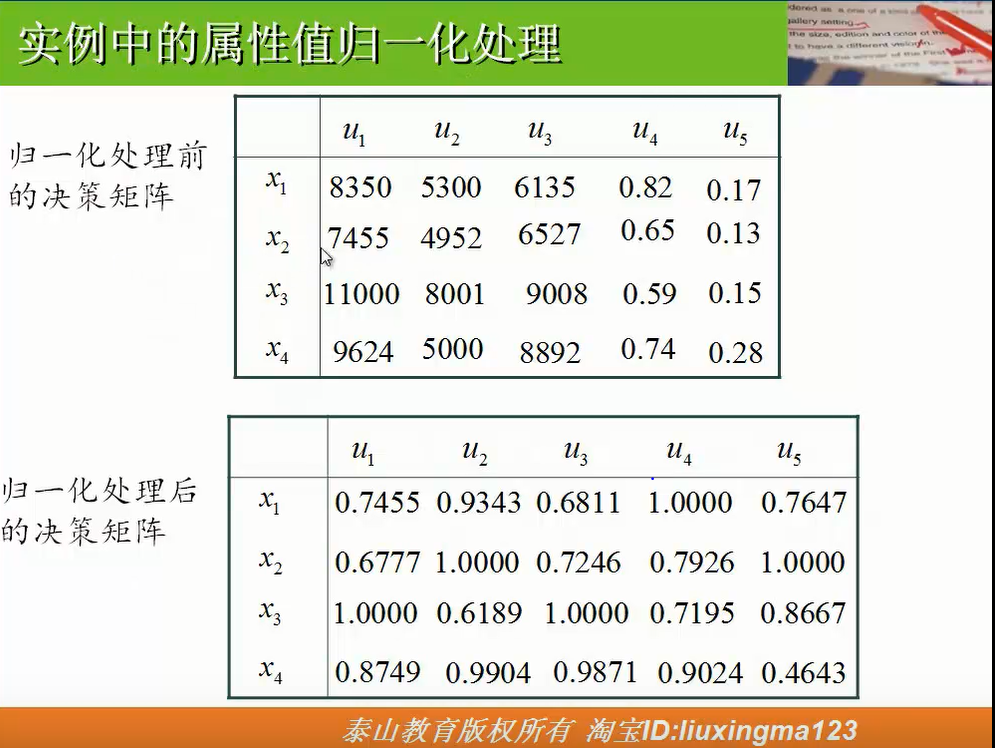利用各项指标权值与每个公司对应数据相乘再相加得到的总值对比。


展开全文• ## 多属性决策

千次阅读 2018-07-27 10:05:16
多属性决策 几个应该注意的要点 关于这一模型其实没有太多要记的，但是有几个点应当了解一下 属性值应该归一化（对于量纲不统一） 对评价指标权重构建成对比较矩阵 用归一化后的属性值乘以对应权重再加和，值...
多属性决策

多属性决策
几个应该注意的要点
属性值的归一化处理
属性类型
归一化公式
效益型
成本型
固定型
偏离型
区间型
偏离区间型

举例

几个应该注意的要点

关于这一模型其实没有太多要记的，但是有几个点应当了解一下

属性值应该归一化（对于量纲不统一）
对评价指标权重构建成对比较矩阵
用归一化后的属性值乘以对应权重再加和，值最大的为最佳选择
权重是通过成对比较矩阵带入代码解得

属性值的归一化处理

这里主要谈一下怎么将属性值归一化

属性类型

属性类型一般有效益型、成本型、固定型、偏离型、区间型、偏离区间型等，其中效益型属性是指属性值越大越好的属性，成本型属性是指属性值越小越好的属性，固定型属性是指属性值越接近某个固定值αi${\alpha }_{i}$$α_i$越好的属性，偏离型属性是指属性值越偏离某个固定值βj${\beta }_{j}$$β_j$越好的属性。区间型属性是指属性值越接近某个固定区间 [qj1${q}_{1}^{j}$$q_1^j$,qj2${q}_{2}^{j}$$q_2^j$]（包括落入该区间）越好的属性，偏离区间型属性是指属性值越偏离某个固定区间 [qj1${q}_{1}^{j}$$q_1^j$,qj2${q}_{2}^{j}$$q_2^j$] 越好的属性。

归一化公式

为了消除不同物理量纲对决策结果的影响，决策时可按下列公式对数据进行规范化处理。

效益型

rij=aijmaxiaij${r}_{ij}=\frac{{a}_{ij}}{ma{x}_{i}\phantom{\rule{thinmathspace}{0ex}}{a}_{ij}}$	r_{ij} = \dfrac{a_{ij}}{max_i\,a_{ij}}
或
rij=aij−minaijmaxiaij−miniaij${r}_{ij}=\frac{{a}_{ij}-min\phantom{\rule{thinmathspace}{0ex}}{a}_{ij}}{ma{x}_{i}\phantom{\rule{thinmathspace}{0ex}}{a}_{ij}-mi{n}_{i}\phantom{\rule{thinmathspace}{0ex}}{a}_{ij}}$	r_{ij} = \dfrac{a_{ij}-min\,a_{ij}}{max_i\,a_{ij}-min_i\,a_{ij}}

成本型

rij=miniaijaij${r}_{ij}=\frac{mi{n}_{i}\phantom{\rule{thinmathspace}{0ex}}{a}_{ij}}{{a}_{ij}}$	r_{ij} = \dfrac{min_i\,a_{ij}}{a_{ij}}
或
rij=maxiaij−aijmaxiaij−miniaij${r}_{ij}=\frac{ma{x}_{i}\phantom{\rule{thinmathspace}{0ex}}{a}_{ij}-{a}_{ij}}{ma{x}_{i}\phantom{\rule{thinmathspace}{0ex}}{a}_{ij}-mi{n}_{i}\phantom{\rule{thinmathspace}{0ex}}{a}_{ij}}$	r_{ij} = \dfrac{max_i\,a_{ij} - a_{ij}}{max_i\,a_{ij} - min_i\,a_{ij}}

固定型

rij=1−aij−αjmaxi|aij−αj|${r}_{ij}=1-\frac{{a}_{ij}-{\alpha }_{j}}{ma{x}_{i}\phantom{\rule{thinmathspace}{0ex}}|{a}_{ij}-{\alpha }_{j}|}$	r_{ij} = 1 - \dfrac{a_{ij} - α_j}{max_i\,\vert{a_{ij} - α_j}\vert}

偏离型

rij=|aij−βj|−mini|aij−βj|maxi|aij−βj|−mini|aij−βj|${r}_{ij}=|{a}_{ij}-{\beta }_{j}|-\frac{mi{n}_{i}\phantom{\rule{thinmathspace}{0ex}}|{a}_{ij}-{\beta }_{j}|}{ma{x}_{i}\phantom{\rule{thinmathspace}{0ex}}|{a}_{ij}-{\beta }_{j}|-mi{n}_{i}\phantom{\rule{thinmathspace}{0ex}}|{a}_{ij}-{\beta }_{j}|}$	r_{ij} = \vert{a_{ij} - β_j}\vert - \dfrac{min_i\,\vert{a_{ij} - β_j}\vert}{max_i\,\vert{a_{ij} - β_j}\vert - min_i\,\vert{a_{ij} - β_j}\vert}

区间型

f(n)=⎧⎩⎨⎪⎪⎪⎪1−max(qj1−aij,aij−qj2)max(qj1−miniaij,maxiaij−qj2),1,aij∉[qj1,qj2]aij∈[qj1,qj2]$f\left(n\right)=\left\{\begin{array}{ll}1-\frac{max\left({q}_{1}^{j}-{a}_{ij},{a}_{ij}-{q}_{2}^{j}\right)}{max\left({q}_{1}^{j}-mi{n}_{i}\phantom{\rule{thinmathspace}{0ex}}{a}_{ij},ma{x}_{i}\phantom{\rule{thinmathspace}{0ex}}{a}_{ij}-{q}_{2}^{j}\right)},& {a}_{ij}\notin \left[{q}_{1}^{j},{q}_{2}^{j}\right]\\ 1,& {a}_{ij}\in \left[{q}_{1}^{j},{q}_{2}^{j}\right]\end{array}$	f(n) =
\begin{cases}
1 - \dfrac{max(q_1^j - a_{ij},a_{ij} - q_2^j)}{max(q_1^j - min_i\,a_{ij},max_i\,a_{ij} - q_2^j)},  & \text{$a_{ij}\notin[q_1^j,q_2^j]$} \\
1, & \text{$a_{ij}\in[q_1^j,q_2^j]$}
\end{cases}

偏离区间型

f(n)=⎧⎩⎨⎪⎪⎪⎪max(qj1−aij,aij−qj2)max(qj1−miniaij,maxiaij−qj2),0,aij∉[qj1,qj2]aij∈[qj1,qj2]$f\left(n\right)=\left\{\begin{array}{ll}\frac{max\left({q}_{1}^{j}-{a}_{ij},{a}_{ij}-{q}_{2}^{j}\right)}{max\left({q}_{1}^{j}-mi{n}_{i}\phantom{\rule{thinmathspace}{0ex}}{a}_{ij},ma{x}_{i}\phantom{\rule{thinmathspace}{0ex}}{a}_{ij}-{q}_{2}^{j}\right)},& {a}_{ij}\notin \left[{q}_{1}^{j},{q}_{2}^{j}\right]\\ 0,& {a}_{ij}\in \left[{q}_{1}^{j},{q}_{2}^{j}\right]\end{array}$	f(n) =
\begin{cases}
\dfrac{max(q_1^j - a_{ij},a_{ij} - q_2^j)}{max(q_1^j - min_i\,a_{ij},max_i\,a_{ij} - q_2^j)},  & \text{$a_{ij}\notin[q_1^j,q_2^j]$} \\
0, & \text{$a_{ij}\in[q_1^j,q_2^j]$}
\end{cases}

举例

一组数值为成本型属性值：5300 4952    8001    5000
归一化处理后得到：0.9343 1.0000  0.6189  0.9904
一组数值为效益型属性值：0.82 0.65 0.59 0.74
归一化处理后得到：1.0000 0.7926 0.7195 0.9024

展开全文数学建模 MATLAB
• 多属性决策模型加权算术平均算子WAA属性类型有：归一化的代码结果真正的加权算术平均算子WAA结果 加权算术平均算子WAA 就是对属性值*对应的权重求和 属性值要进行归一化处理（标准要一样） 属性类型有： 效益型属性 ...
多属性决策模型加权算术平均算子WAA属性类型有：归一化的代码结果真正的加权算术平均算子WAA结果
加权算术平均算子WAA
就是对属性值*对应的权重求和
属性值要进行归一化处理（标准要一样）
属性类型有：
效益型属性
成本型属性
固定值属性
偏离型属性
区间型属性
原始属性值–>归一化处理后的属性值–>各属性的权重用层次分析法–>得到各项的加权算术平均算子
取最大的投资
归一化的代码
disp('请输入');
x = input('x(i) = ');
len = length(x);
a = max(x);
b = min(x);
disp('效益型:输入1；成本型:输入2');
c = input('options = ');
if c == 1
for i = 1: len
x(i) = x(i)/a;
end
else
for i = 1:len
x(i) = b/x(i);
end
end
x

结果绝大部分都只是效益型和成本型，所以只写了这两种
真正的加权算术平均算子WAA
%%%  归一化后所得数据为u矩阵
u = [0.7591    0.6777    1.0000    0.8749;
0.9343    1.0000    0.6189    0.9904;
0.6811    0.7246    1.0000    0.9871;
1.0000    0.7927    0.7195    0.9024;
0.7647    1.0000    0.8667    0.4643];

%u(7) = 1.0000  %它是竖着数的
[m,n] = size(u); % hang m = 5  lie n = 4(种类）

% 权重比是自己输入的成对比较矩阵用层次分析法算出来的
w = [0.4286
0.1429
0.1429
0.1429
0.1429];

s = zeros(1,n);
for j = 1:n
for i = 1:m
s(j) = s(j) + u(i + (j - 1)*m)*w(i);
end
end
s

结果所以选第三个，因为第三个的权重最大
详细解说见B站


展开全文• 文章目录一、算法介绍1. 加权算术平均算子（WAA）2. 属性值归一化处理二、适用问题三、算法总结1. 步骤四、应用场景举例（企业评估）1...  多属性决策是现代决策科学的一个重要组成部分，它的理论和方法在工程设计、经
文章目录一、算法介绍1. 加权算术平均算子（WAA）2. 属性值归一化处理二、适用问题三、算法总结1. 步骤四、应用场景举例（企业评估）1. 建模构建决策矩阵2. 属性值归一化3. 对各个属性构造成对比较矩阵计算属性权重（层次分析法）4. 计算每个公司的WAA五、MATLAB代码六、实际案例七、论文案例片段（待完善）

多属性决策模型主要针对数学建模问题中的一些小的子问题进行求解，如果想直接使用请跳转至——四、五
视频回顾

一、算法介绍
多属性决策是现代决策科学的一个重要组成部分，它的理论和方法在工程设计、经济、管理和军事等诸多领域中有着广泛的应用，如:投资决策、项目评估、维修服务、武器系统性能评定、工厂选址、投标招标、产业部门发展排序和经济效益综合评价等.
多属性决策的实质是利用已有的决策信息通过一定的方式对一组(有限个)备选方案进行排序或择优.它主要由两部分组成:
(1)获取决策信息．决策信息一般包括两个方面的内容：属性权重和属性值。
(2)通过一定的方式对决策信息进行集结并对方案进行排序和择优。信息集结方法有很多，本节只用加权算术平均(WAA)算子，就相当于计算加权平均数。
1. 加权算术平均算子（WAA）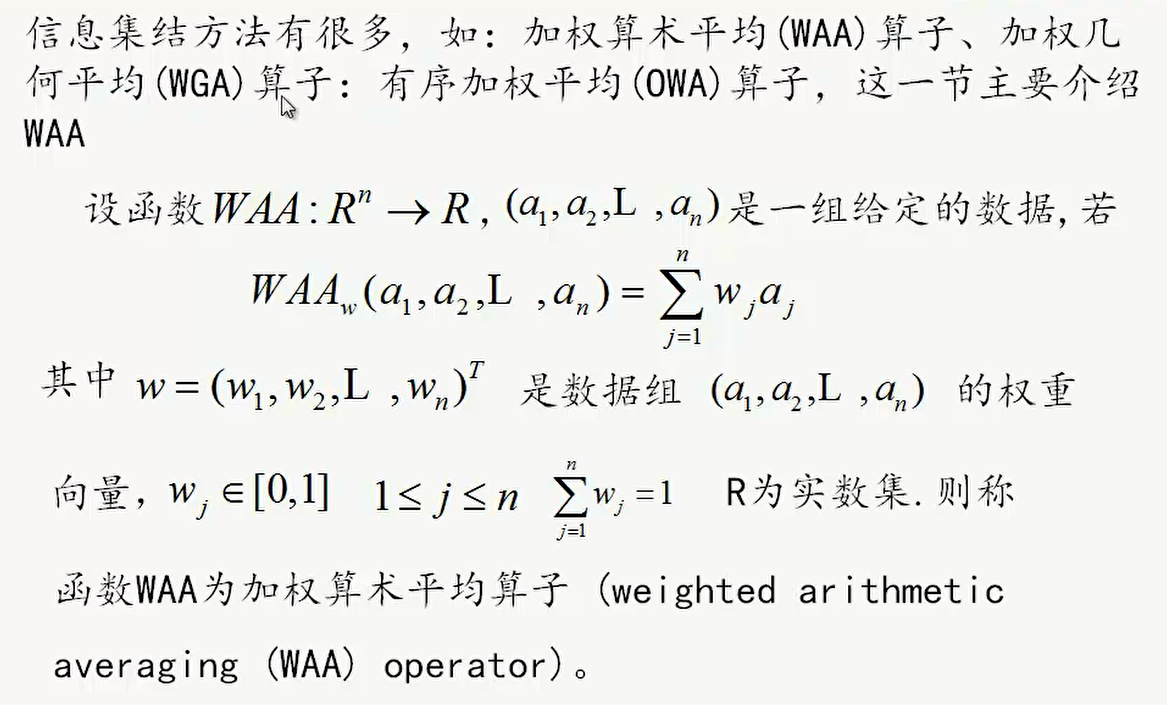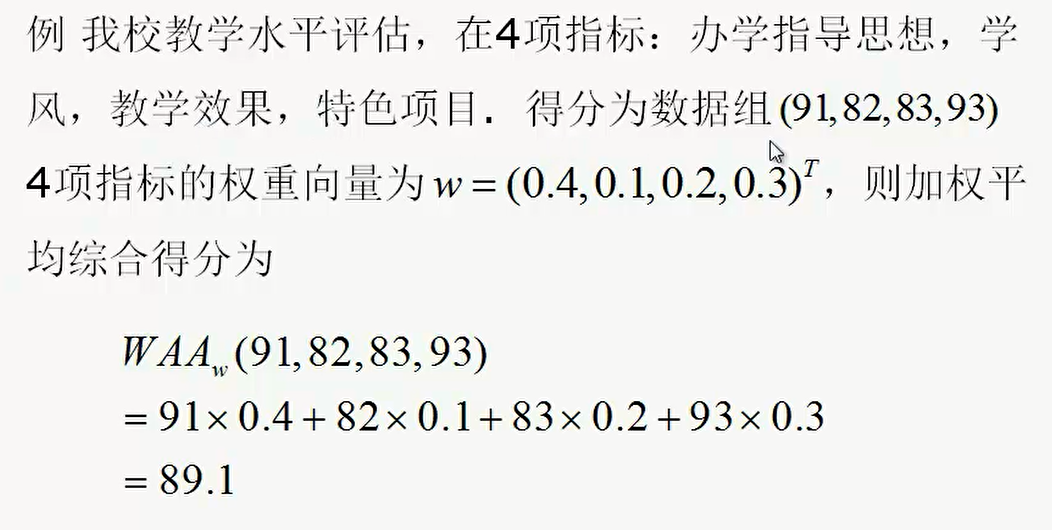2. 属性值归一化处理效益型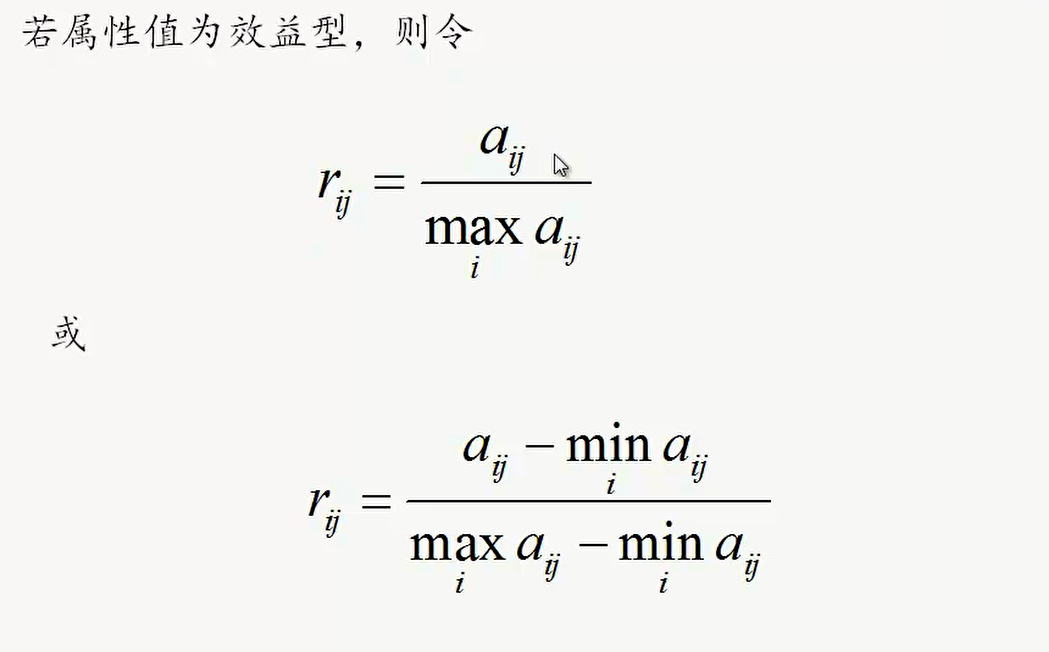成本型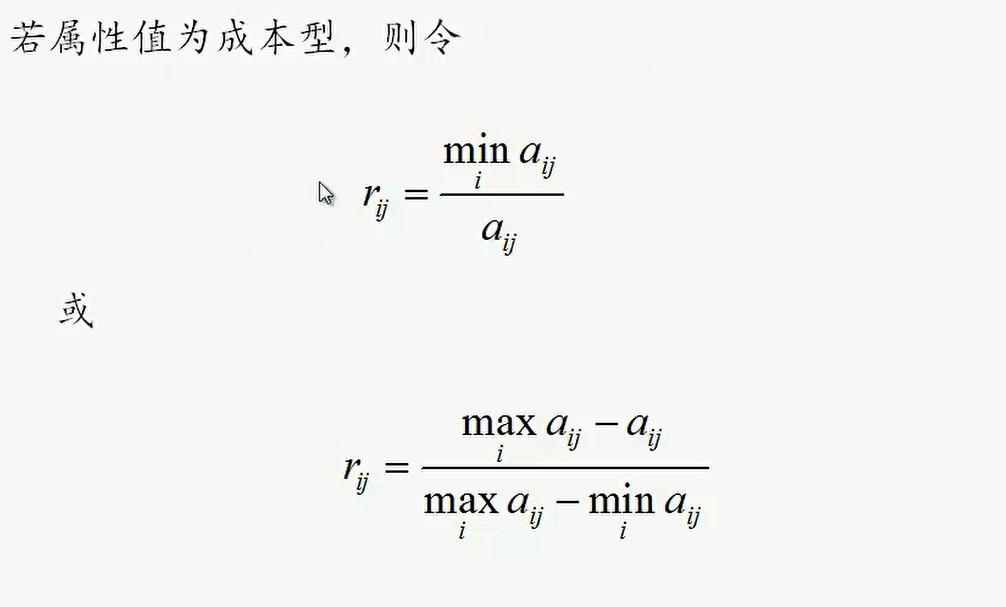固定型、偏离型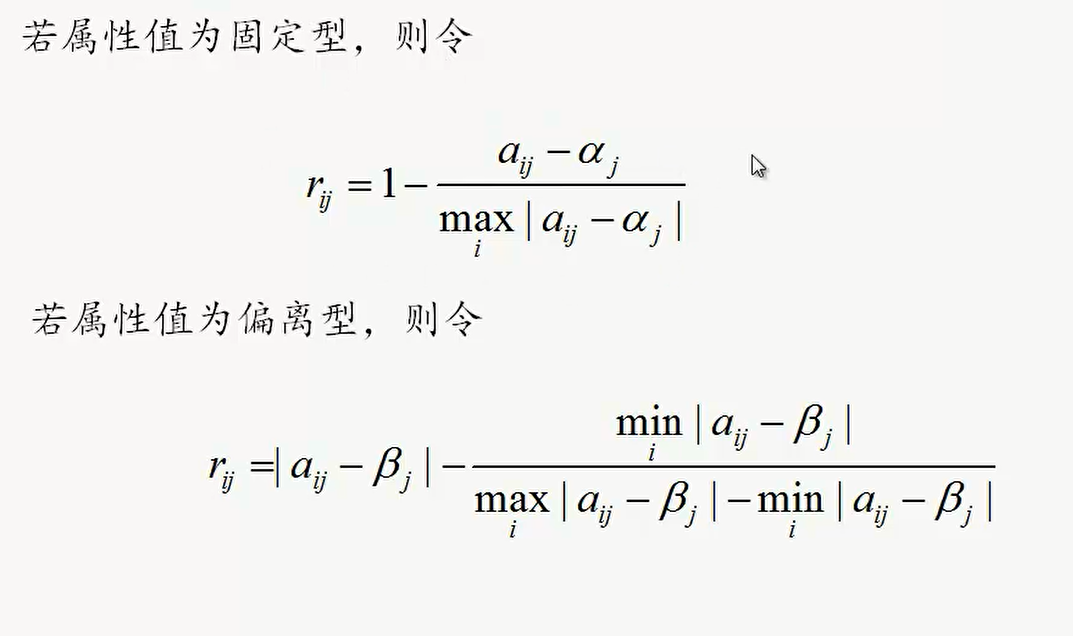区间型、偏离区间型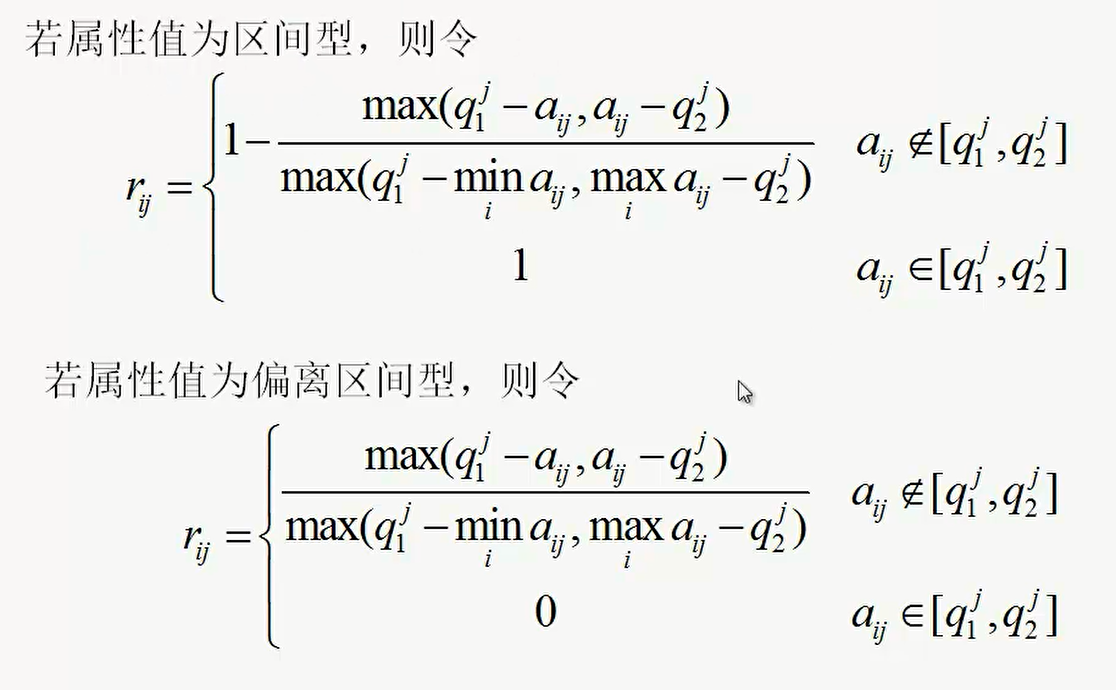二、适用问题

面临多种方案时,根据多个属性及一定的标准选择某一种方案：
例如：

银行根据多个属性对公司进行评估

三、算法总结
1. 步骤

建模构建决策矩阵
属性值归一化
对各个属性构造成对比较矩阵计算属性权重
计算每个公司的WAA

四、应用场景举例（企业评估）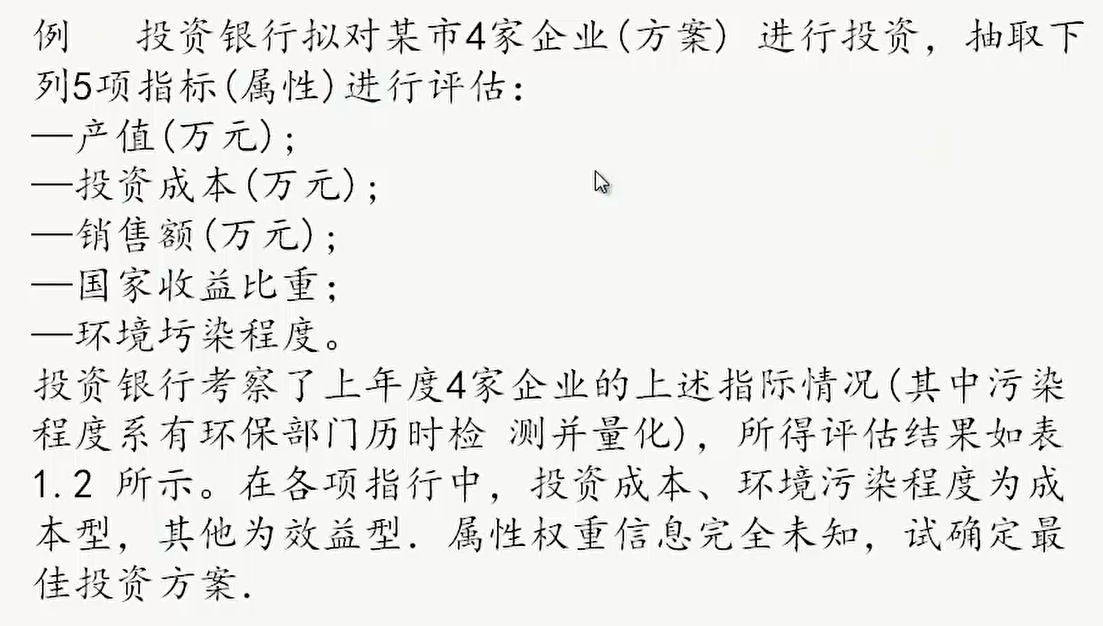1. 建模构建决策矩阵
划分各类属性指标的类型，并构建决策矩阵
2. 属性值归一化
根据属性指标类型，代入公式，进行归一化处理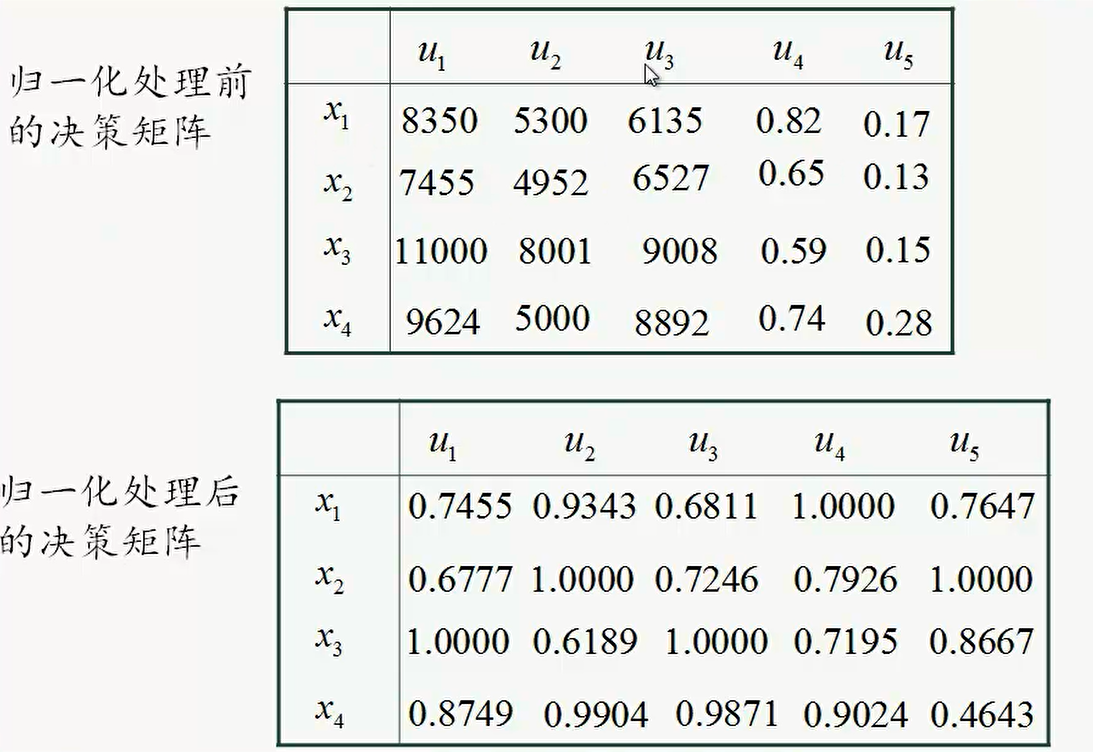3. 对各个属性构造成对比较矩阵计算属性权重（层次分析法）
可以参考层次分析法进行属性权重计算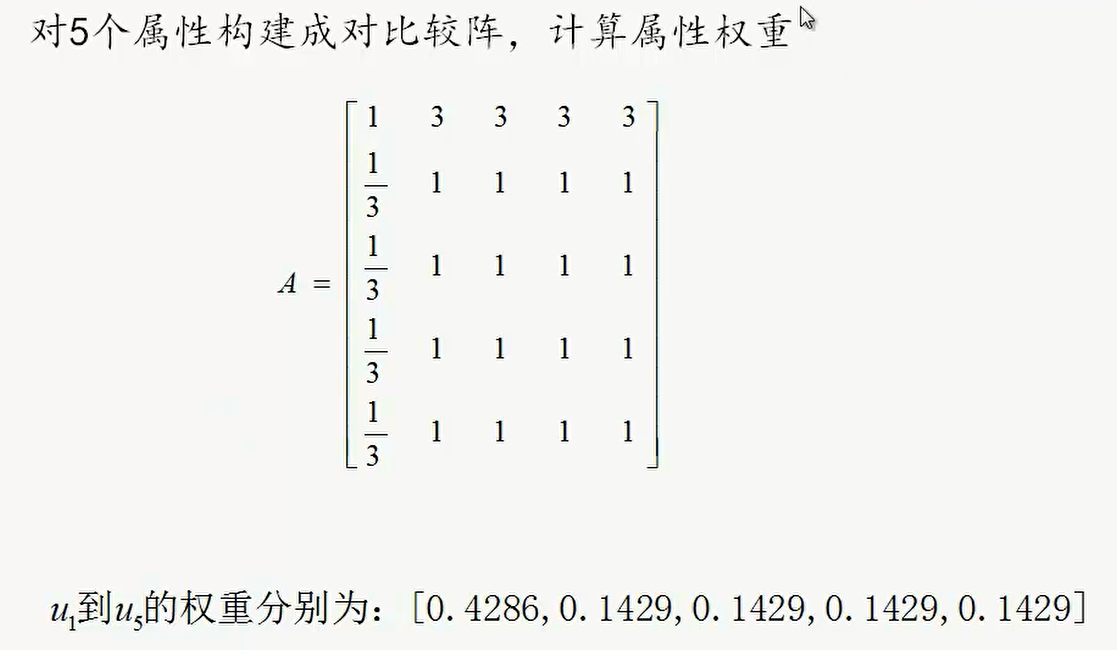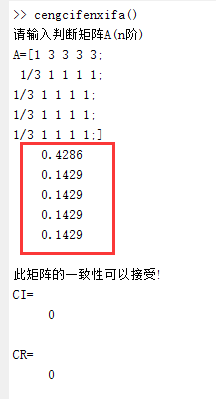4. 计算每个公司的WAA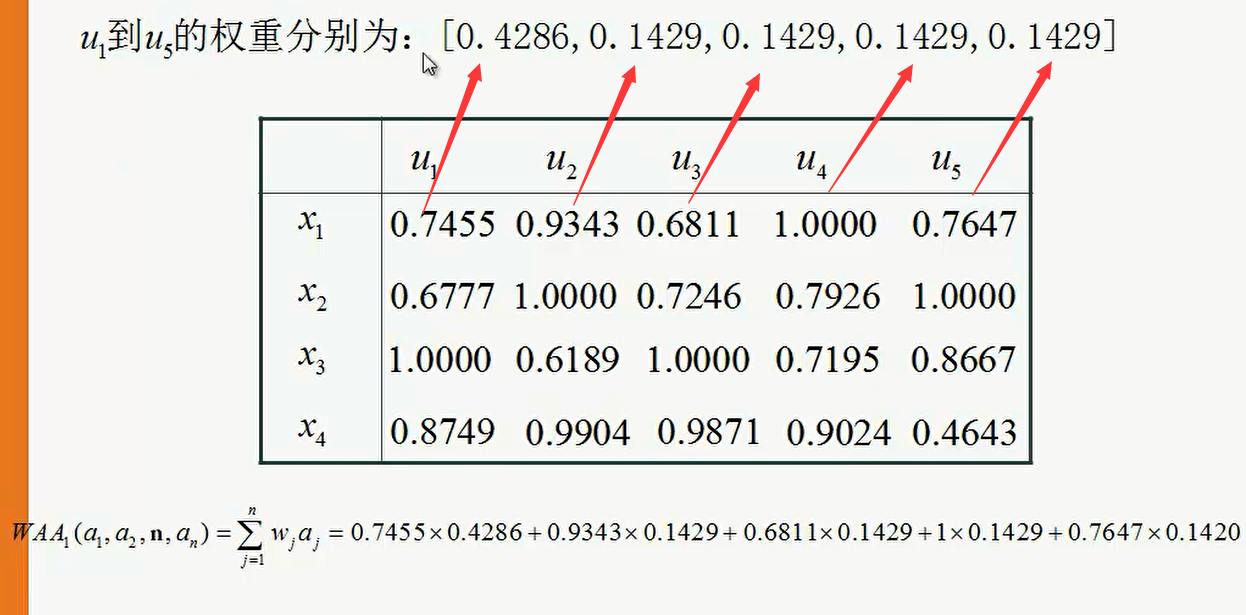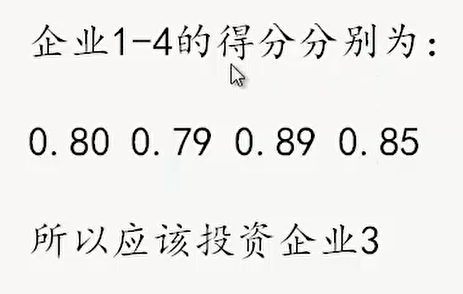五、MATLAB代码
代码是层次分析法的
disp('请输入判断矩阵A(n阶)');
A=input('A=');
[n,n]=size(A);
x=ones(n,100);
y=ones(n,100);
m=zeros(1,100);
m(1)=max(x(:,1));
y(:,1)=x(:,1);
x(:,2)=A*y(:,1);
m(2)=max(x(:,2));
y(:,2)=x(:,2)/m(2);
p=0.0001;i=2;k=abs(m(2)-m(1));
while  k>p
i=i+1;
x(:,i)=A*y(:,i-1);
m(i)=max(x(:,i));
y(:,i)=x(:,i)/m(i);
k=abs(m(i)-m(i-1));
end
a=sum(y(:,i));
w=y(:,i)/a;
t=m(i);
disp(w);
%以下是一致性检验
CI=(t-n)/(n-1);RI=[0 0 0.52 0.89 1.12 1.26 1.36 1.41 1.46 1.49 1.52 1.54 1.56 1.58 1.59];
CR=CI/RI(n);
if CR<0.10
disp('此矩阵的一致性可以接受!');
disp('CI=');disp(CI);
disp('CR=');disp(CR);
end


六、实际案例
[1 3 3 3 3;
1/3 1 1 1 1;
1/3 1 1 1 1;
1/3 1 1 1 1;
1/3 1 1 1 1;]

七、论文案例片段（待完善）


展开全文人工智能 机器学习 算法
• 文章目录：加权算术平均算子属性化的归一化处理多属性决策模型实例 加权算术平均算子 属性化的归一化处理 多属性决策模型实例 首先我们要判断u1～u5的属性值都对应着什么。一般而言，我们的属性值要么是...
• MATLAB和JAGS代码可用于推断多属性选择任务中决策策略中的切换。 使用的所有分析和软件包。 代码 两个主要代码文件是用于分层模型的MATLAB脚本strategySwitchingOneGroup.m和用于潜在混合物扩展的...
• 文章目录算法简介调用示例例题主函数代码结果具体实现准备函数Step1:指标数据的三角形模糊数表达Step2: 模糊指标矩阵 F 归一化处理Step3: 构造模糊决策矩阵Step4: 确定模糊正理想 M + 与模糊负理想 M −Step5:确定...
• 这是李登峰老师《直觉模糊集决策与对策分析方法》第二章直觉模糊集合多属性决策的直觉模糊广义混合加权集结方法。 对于给出的算例进行独立重复实验。开头的注释是我学习过程中遇到的insert函数的使用方法，之后的...
• 例如，根据图1的决策树模型，运用自顶而下的属性测试过程，将表2中的样例1-6分别分类为“Y”、“Y”、“Y”、“Y”、“N”、“N”。 图1. 一个决策树模型的例子 (3) 举例：对下表运用算法构建决策树 表1. 一个训练...
• matlab代码多使用从头开始的决策树进行分类 介绍 利用决策树基于ID3算法对字母识别数据进行分类，得到混淆矩阵。 第一部分 该树是根据信息增益（IG）准则进行训练的。 第二部分 该树是根据基尼系数准则进行训练的。 ...
• 决策树的本质是一棵由个判断节点组成的树，其中每个内部节点表示一个属性上的判断，每个分支代表一个判断结果的输出，最后每个叶节点代表一种分类结果。 思考：如果一个训练数据集，包含个特征，在构造决策树的...
• 基础算法 举个栗子： 当一个有经验的老农看一个瓜是不是好瓜时，他可能会先看下瓜的颜色，一看是青绿的，心想有可能是好瓜！接着他又看了下根蒂，发现是...代码的形式表示： 划分属性选择 上图算法最重要的...剪枝算法 C4.5算法 CART算法
• ## 多变量决策树

千次阅读 热门讨论 2020-06-02 19:23:15
本文使用PyTorch进行变量决策树的实现. 变量决策树的结构为二叉树，实际上是个线性模型的组合，原理可见下图： 图4.13表示的是变量决策树的结构，图4.14表示的是对应的分类边界 代码实现 定义数据集 首先将...机器学习 算法 python
• 每个决策节点表示一个待分类的数据类别或属性，每个叶节点表示一种结果。整个决策的过程从根决策节点开始，从上到下。根据数据的分类在每个决策节点给出不同的结果。 决策树包含很算法，下面介绍一下常见的ID3、C...
• 变量决策树1 变量决策树简介2 实现思路3 代码中的函数说明3.1 class TreeNode3.2 trainLinear3.3 binaryTrainSet3.4 score3.5 treeGenerate3.6 predict3.7 evaluate4 完整代码5 结果 1 变量决策树简介   ...机器学习 python 数据分析
• 一、决策决策树学习是机器学习中一类常用的算法。在决策树中，根节点包含样本全集。每个非叶子节点表示一种对样本的分割，...划分属性一般采用样本的某个特征或个特征的组合。决策树学习是一种有监督学习，根据样随机森林 机器学习
• C4.5算法较之ID3算法主要有4点改进： 采用信息增益率作为最优划分属性。 能够处理连续值类型的属性。 能够处理缺失值属性。...ID3算法中的信息增益准则对取值数目较属性有所偏好，例如西瓜数据集中，如果把“...机器学习
• 好吧，谈谈今日缝合怪之粗糙集属性约简+ID3决策树，用的是之前的UCI DNA数据集，基本上就是之前ID3决策树改了改。 （代码晚点贴，目前仅讲讲思路） 先贴UCI DNA数据集：...机器学习 人工智能
• 该存储库包含用于几种机器学习算法的R代码：SVM，PerTurbo，朴素贝叶斯，项逻辑回归，决策树和随机森林。 它还具有读取和写入Envi格式（二进制文件）的遥感图像以及执行统计显着性检验的功能。 有关运行代码，请...
• 这是我最喜欢的算法之一，我经常使用它。...有关更详细信息，请参阅： 简化决策树。 在上图中，您可以看到人口根据属性分为四个不同的组，以确定 “ 他们是否会玩 ”。为了将群体分成不同的异构群体...机器学习
• 叶结点对应于决策结果，其他每个结点对应于一个属性测试。 每个结点包含的样本根据属性测试的结果被划分到子节点当中。 根节点包含样本全集。 决策树学习的目的是为了产生一棵泛化能力强，即处理未见示例能力强的...
• 是一种树形结构，其中每个内部节点表示一个属性上的判断，每个分支代表一个判断结果的输出最后每个叶节点代表一种分类结果，本质是一颗由个判断节点组成的树 信息熵 熵 用于衡量一个对象的有序程度 从信息的...python 机器学习
• 机器学习项目实战----基于鸢尾花数据集（python代码，多种算法对比：决策树、SVM、k近邻） 1、项目介绍 鸢尾花数据集是机器学习入门的一个十分经典的数据集，这里依然简单的介绍一下这个项目是做什么的，通过花萼...机器学习 支持向量机 k近邻
• 文章目录综合评价与决策方法理想解法定义及有关概念TOPSIS法...多属性决策问题的理想解法，亦称TOPSIS法，即各指标的最优解和最劣解。 多属性决策方案集DDD={d1,d2,…,dmd_1,d_2,…,d_md1​,d2​,…,dm​} 衡量方案优劣
• AHP代码有助于解决多目标多属性决策问题，简单实用，方便好操作，是一matlab为平台，进行编辑的。
• 实体为人,他有很多属性,长相,身高…. 信息: 属性的特征 :长相(属性): 很帅(特征) 熵: (包含所有的属性的信息(特征): 身高:很高,长相:很帅,经济:很有钱) 对它进行求熵 条件熵: 某个属性的信息(特征),求熵(如:身高...
• 2、C4.5算法划分（为了解决ID3算法对取值数目较属性有所偏好的问题） 增益率最大的属性做为最有划分属性 3、基尼系数划分 基尼系数最小的属性做为最有划分属性 剪枝处理防止过拟合 1、预剪枝 （树生成的过程中...算法 python
• 决策树和随机森林都是常用的分类算法，它们的判断逻辑和人的思维方式非常类似，人们常常在遇到个条件组合问题的时候，也通常可以画出一颗决策树来帮助决策判断。本文简要介绍了决策树和随机森林的算法以及实现，并...
• 这里写目录标题决策代码运行结果决策决策决策树（Decision Tree）是一种基本的分类与回归方法，当决策树用于分类时称为分类树，用于回归时称为回归树。主要介绍分类树。 决策树由结点和有向边组成。结点...
• 决策树的思想比较简单，不复杂，决策树，就是通过一个属性将数据进行划分，而这个属性的选择也就是决策树的关键，用什么样的属性分开的值尽可能属于同一个类别。 属性选择的方法很，书中主要介绍了：通过信息增益......